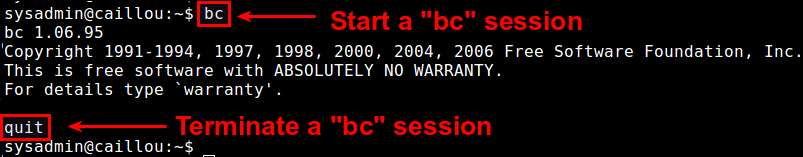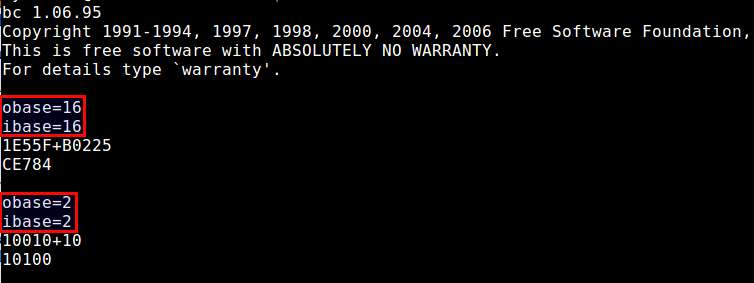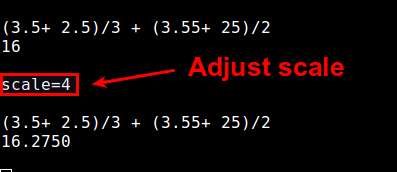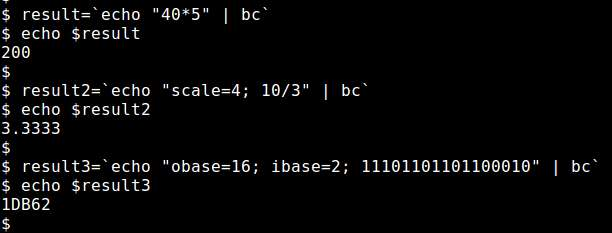# What is a good command-line calculator on Linux

Last updated on August 14, 2020 by Dan Nanni

Every modern Linux desktop distribution comes with a default GUI-based calculator app. On the other hand, if your workspace is full of terminal windows, and you would rather crunch some numbers within one of those terminals quickly, you are probably looking for a command-line calculator. In this category, GNU `bc` (short for "basic calculator") is a hard to beat one. While there are many command-line calculators available on Linux, I think GNU `bc` is hands-down the most powerful and useful.

Predating the GNU era, `bc` is actually a historically famous arbitrary precision calculator language, with its first implementation dating back to the old Unix days in 1970s. Initially `bc` was a better known as a programming language whose syntax is similar to C language. Over time the original `bc` evolved into POSIX `bc`, and then finally GNU `bc` of today.

## Features of GNU `bc`

Today's GNU `bc` is a result of many enhancements of earlier implementations of `bc`, and now it comes standard on all major GNU/Linux distros. It supports standard arithmetic operators with arbitrary precision numbers, and multiple numeric base (e.g., binary, decimal hexadecimal) of input and output.

If you are familiar with C language, you will see that the same or similar mathematical operators are used in `bc`. Some of supported operators include arithmetic (+, -, *, /, %, ++, --), comparison (<, >, ==, !=, <=, >=), logical (!, &&, ||), bitwise (&, |, ^, ~, <<, >>), compound assignment (+=, -=, *=, /=, %=, &=, |=, ^=, &&=, ||=, <<=, >>=) operators. `bc` comes with many useful built-in functions such as square root, sine, cosine, arctangent, natural logarithm, exponential, etc.

## How to Use GNU `bc`

As a command-line calculator, possible use cases of GNU `bc` are virtually limitless. In this tutorial, I am going to describe a few popular features of `bc` command. For a complete manual, refer to the official source.

Unless you have a pre-written `bc` script, you typically run `bc` in interactive mode, where any typed statement or expression terminated with a newline is interpreted and executed on the spot. Simply type the following to enter an interactive `bc` session. To quit a session, type '`quit`' and press Enter.

```\$ bc
```The examples presented in the rest of the tutorial are supposed to be typed inside a `bc` session.

### Type Expressions

To calculate an arithmatic expression, simply type the expression at the blinking cursor, and press `ENTER`. If you want, you can store an intermediate result to a variable, then access the variable in other expressions.Within a given session, `bc` maintains a unlimited history of previously typed lines. Simply use UP arrow key to retrieve previously typed lines. If you want to limit the number of lines to keep in the history, assign that number to a special variable named `history`. By default the variable is set to -1, meaning unlimited.

### Switch Input/Output Base

Often times you want to type input expressions and display results in binary or hexadecimal formats. For that, `bc` allows you switch the numeric base of input or output numbers. Input and output bases are stored in `ibase` and `obase`, respectively. The default value of these special variables is 10, and valid values are 2 through 16 (or the value of `BC_BASE_MAX` environment variable in case of `obase`). To switch numeric base, all you have to do is to change the values of `ibase` and `obase`. For example, here are examples of summing up two hexadecimal/binary numbers:Note that here I specify `obase=16` before `ibase=16`, not vice versa. That is because if I specified `ibase=16` first, the subsequent `obase=16` statement would be interpreted as assigning 16 in base 16 to `obase` (i.e., 22 in decimal), which is not what we want.

### Adjust Precision

In `bc`, the precision of numbers is stored in a special variable named `scale`. This variable represents the number of decimal digits after the decimal point. By default, `scale` is set to 0, which means that all numbers and results and truncated/stored in integers. To adjust the default precision, all you have to do is to change the value of `scale` variable.

```scale=4
```### Use Built-in Functions

Beyond simple arithmatic operations, GNU `bc` offers a wide range of advanced mathematical functions built-in, via an external math library. To use those functions, launch `bc` with `-l` option from the command line.

Some of these built-in functions are illustrated here.

#### 1. Square root of `N`:

```sqrt(N)
```

```s(X)
```

```c(X)
```

```a(X)
```

```l(X)
```

```e(X)
```

### Other Goodies as a Language

As a full-blow calculator language, GNU `bc` supports simple statements (e.g., variable assignment, break, return), compound statements (e.g., if, while, for loop), and custom function definitions. I am not going to cover the details of these features, but you can easily learn how to use them from the official manual. Here is a very simple function definition example:

```define dummy(x){
return(x * x);
}
dummy(9)
81
dummy(4)
16
```

### Use GNU `bc` Non-interactively

So far we have used `bc` within an interactive session. However, quite popular use cases of `bc` in fact involve running `bc` within a shell script non-interactively. In this case, you can send input to `bc` using `echo` through a pipe. For example:

```\$ echo "40*5" | bc
\$ echo "scale=4; 10/3" | bc
\$ echo "obase=16; ibase=2; 11101101101100010" | bc
```## Conclusion

To conclude, GNU `bc` is a powerful and versatile command-line calculator that really lives up to your expectation. Preloaded on all modern Linux distributions, `bc` can make your number crunching tasks much easy to handle without leaving your terminals. Besides, if your shell script requires floating point calculation, `bc` can easily be invoked by the script to get the job done. All in all, GNU `bc` should definitely be in your productivity toolset.

## Support Xmodulo

This website is made possible by minimal ads and your gracious donation via PayPal (Credit Card) or Bitcoin (`1M161JGAkz3oaHNvTiPFjNYkeABox8rb4g`).

Please note that this article is published by Xmodulo.com under a Creative Commons Attribution-ShareAlike 3.0 Unported License. If you would like to use the whole or any part of this article, you need to cite this web page at Xmodulo.com as the original source.

Xmodulo © 2021 ‒ AboutWrite for UsFeed ‒ Powered by DigitalOcean